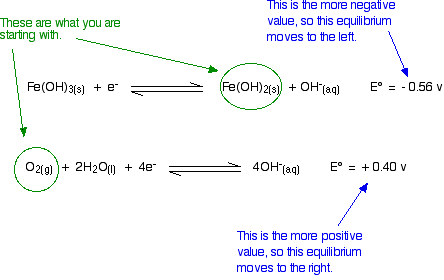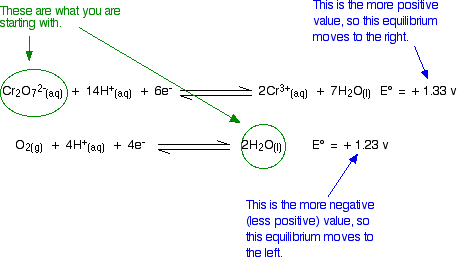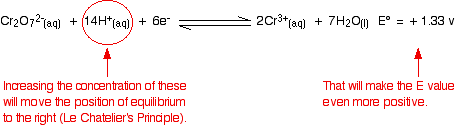# Using Redox Potentials to Predict the Feasibility of Reactions

$$\newcommand{\vecs}{\overset { \rightharpoonup} {\mathbf{#1}} }$$ $$\newcommand{\vecd}{\overset{-\!-\!\rightharpoonup}{\vphantom{a}\smash {#1}}}$$$$\newcommand{\id}{\mathrm{id}}$$ $$\newcommand{\Span}{\mathrm{span}}$$ $$\newcommand{\kernel}{\mathrm{null}\,}$$ $$\newcommand{\range}{\mathrm{range}\,}$$ $$\newcommand{\RealPart}{\mathrm{Re}}$$ $$\newcommand{\ImaginaryPart}{\mathrm{Im}}$$ $$\newcommand{\Argument}{\mathrm{Arg}}$$ $$\newcommand{\norm}{\| #1 \|}$$ $$\newcommand{\inner}{\langle #1, #2 \rangle}$$ $$\newcommand{\Span}{\mathrm{span}}$$ $$\newcommand{\id}{\mathrm{id}}$$ $$\newcommand{\Span}{\mathrm{span}}$$ $$\newcommand{\kernel}{\mathrm{null}\,}$$ $$\newcommand{\range}{\mathrm{range}\,}$$ $$\newcommand{\RealPart}{\mathrm{Re}}$$ $$\newcommand{\ImaginaryPart}{\mathrm{Im}}$$ $$\newcommand{\Argument}{\mathrm{Arg}}$$ $$\newcommand{\norm}{\| #1 \|}$$ $$\newcommand{\inner}{\langle #1, #2 \rangle}$$ $$\newcommand{\Span}{\mathrm{span}}$$$$\newcommand{\AA}{\unicode[.8,0]{x212B}}$$

This page explains how to use redox potentials (electrode potentials) to predict the feasibility of redox reactions. It also looks at how you go about choosing a suitable oxidizing agent or reducing agent for a particular reaction.

## Predicting the Feasibility of a Possible Redox reaction

Standard electrode potentials (redox potentials) are one way of measuring how easily a substance loses electrons. In particular, they give a measure of relative positions of equilibrium in reactions such as:

$Zn^{2+} + 2e^- \rightleftharpoons Zn (s) \nonumber$

with $$E^o = -0.76 \,V$$ and

$Cu^{2+} + 2e^- \rightleftharpoons Cu (s) \nonumber$

with $$E^o = +0.34 \,V$$.

The more negative the E° value, the further the position of equilibrium lies to the left. Remember that this is always relative to the hydrogen equilibrium - and not in absolute terms. The negative sign of the zinc E° value shows that it releases electrons more readily than hydrogen does. The positive sign of the copper E° value shows that it releases electrons less readily than hydrogen.

The more negative the E° value, the further the position of equilibrium lies to the left of a standard reduction half reaction.

Whenever you link two of these equilibria together (either via a bit of wire, or by allowing one of the substances to give electrons directly to another one in a test tube) electrons flow from one equilibrium to the other. That upsets the equilibria and Le Chatelier's principle applies to figure out how new equilibria are established. The positions of equilibrium move - and keep on moving if the electrons continue to be transferred.

The two equilibria essentially turn into two one-way reactions:

• The equilibrium with the more negative (or less positive) E° value will move to the left.
• The equilibrium with the more positive (or less negative) E° value will move to the right.
##### 4

Will magnesium react with dilute sulfuric acid?

###### Solution

The relevant reduction reactions and associated potentials (via Table P2) for this system are

$Mg^{2+} (aq) + 2e^- \rightleftharpoons Mg (s) \nonumber$

with $$E^o = -2.37 \,V$$ and

$S_2O_8^{2−} + 2e^− \rightleftharpoons 2SO_4^{2−} \nonumber$

with $$E^o = +1.96 \,V$$ and since we are in an aqueous solvent

$2H^{+} (aq) + 2e^- \rightleftharpoons H_2 (g) \nonumber$

with $$E^o = 0 \,V$$.

The sulfate ions are spectator ions and play no part in the reaction; you are essentially starting with magnesium metal and hydrogen ions in the acid.Is there anything to stop the sort of movements we have suggested? No! The magnesium can freely turn into magnesium ions and give electrons to the hydrogen ions producing hydrogen gas.

The reaction is feasible.

Now for a reaction which turns out not to be feasible . .

##### Example $$\PageIndex{2}$$: Copper and Sulfuric acid

Will copper react with dilute sulfuric acid?

###### Solution

You probably know that the answer is that it will not. How do the E° values predict this? The relevant reduction reactions and associated potentials (via Table P2) for this system are

$Cu^{2+} (aq) + 2e^- \rightleftharpoons Cu (s) \nonumber$

with $$E^o = +0.34 \,V$$ and since we are in an aqueous solvent

$2H^{+} (aq) + 2e^- \rightleftharpoons H_2 (g) \nonumber$

with $$E^o = 0 \,V$$.

Doing the same sort of thinking as in Example $$\PageIndex{1}$$:The diagram shows the way that the E° values are telling us that the equilibria will tend to move. Is this possible? No!

• If we start from copper metal, the copper equilibrium is already completely to the right. If it were to react at all, the equilibrium will have to move to the left - directly opposite to what the E° values are saying.
• Similarly, if we start from hydrogen ions (from the dilute acid), the hydrogen equilibrium is already as far to the left as possible. For it to react, it would have to move to the right - against what the E° values demand.

There is no possibility of a reaction.

In the next couple of examples, decide for yourself whether or not the reaction is feasible before you read the text.

##### Example $$\PageIndex{3}$$: Iron and Hydroxide Ions

Will oxygen oxidize iron(II) hydroxide to iron(III) hydroxide under alkaline conditions?

###### Solution

The relevant reduction reactions and associated potentials (via Table P2) for this system are

$Fe(OH)_3 (s) + e^- \rightleftharpoons Fe(OH)_2 (s) + OH^- (aq) \nonumber$

with $$E^o = -0.56 \,V$$ and

$O_2 (g) + 2H_2O (l) \rightleftharpoons 4 OH^- (aq) \nonumber$

with $$E^o = 0.40 \,V$$.

Think about this before you read on. Remember that the equilibrium with the more negative E° value will tend to move to the left. The other one tends to move to the right. Is that possible?Yes, it is possible. Given what we are starting with, both of these equilibria can move in the directions required by the E° values.

The reaction is feasible.

##### Example $$\PageIndex{4}$$

Will chlorine oxidize manganese (II) ions to manganate (VII) ions in acidic solutions?

###### Solution

The relevant reduction reactions and associated potentials (via Table P2) for this system are

$MnO_4^- (aq) + 8H^+(aq) + 5e^- \rightleftharpoons Mn^{2+} (aq) + 4H_2O (l) \nonumber$

with $$E^o = +1.51 \,V$$ and

$Cl_2 (g) + 2e^- \rightleftharpoons 2Cl^- (aq) \nonumber$

with $$E^o = +1.36 \,V$$.Given what you are starting from, these equilibrium shifts are impossible. The manganese equilibrium has the more positive E° value and so will tend to move to the right. However, because we are starting from manganese(II) ions, it is already as far to the right as possible. In order to get any reaction, the equilibrium would have to move to the left. That is against what the E° values are saying.

This reaction is not feasible.

##### Example $$\PageIndex{5}$$: Copper and Nitric Acid

Will dilute nitric acid react with copper?

###### Solution

This is going to be more complicated because there are two different ways in which dilute nitric acid might possibly react with copper. The copper might react with the hydrogen ions or with the nitrate ions. Nitric acid reactions are always more complex than the simpler acids like sulfuric or hydrochloric acid because of this problem.

The relevant reduction reactions and associated potentials (via Table P2) for this system are

$Cu^{2+}(aq) + 2e^- \rightleftharpoons Cu (aq) \nonumber$

with $$E^o = +0.34 \,V$$ and

$2H^{+} (aq) + 2e^- \rightleftharpoons H_2 (g) \nonumber$

with $$E^o = 0 \,V$$ and

$NO_3^{-}(aq) + 4H^+ + 3e^- \rightleftharpoons NO(g) + 2H_2O(l) \nonumber$

with $$E^o = +0.96 \,V$$.

We have already discussed the possibility of copper reacting with hydrogen ions in Example $$\PageIndex{2}$$. Go back and look at it again if you need to, but the argument (briefly) goes like this: The copper equilibrium has a more positive E° value than the hydrogen one. That means that the copper equilibrium will tend to move to the right and the hydrogen one to the left. However, if we start from copper and hydrogen ions, the equilibria are already as far that way as possible. Any reaction would need them to move in the opposite direction to what the E° values want. The reaction isn't feasible.

What about a reaction between the copper and the nitrate ions? This is feasible. The nitrate ion equilibrium has the more positive E° value and will move to the right. The copper E° value is less positive. That equilibrium will move to the left. The movements that the E° values suggest are possible, and so a reaction is feasible.

Copper (II) ions are produced together with nitrogen monoxide gas.

## Using E° Values may give the Incorrect Answer

It sometimes happens that E° values suggest that a reaction ought to happen, but it does not. Occasionally, a reaction happens although the E° values seem to be the wrong way around. These next two examples explain how that can happen. By coincidence, both involve the dichromate(VI) ion in potassium dichromate(VI).

##### Example $$\PageIndex{6}$$: Potassium Dichromate and Water

Will acidified potassium dichromate(VI) oxidize water?

###### Solution

The relevant reduction reactions and associated potentials (via Table P2) for this system are

$Cr_2O_7^{2-} (aq) + 14H^+(aq) + 6e^- \rightleftharpoons 2Cr^{3+} (aq) + 7H_2O (l) \nonumber$

with $$E^o = +1.33 \,V$$ and

$O_2 (g) + 4H^+(aq) + 4e^- \rightleftharpoons 2H_2O (l) \nonumber$

with $$E^o = +1.23 \,V$$.

The relative sizes of the E° values show that the reaction is feasible:However, in the test tube nothing happens however long you wait. An acidified solution of potassium dichromate(VI) does not react with the water that it is dissolved in. So what is wrong with the argument?

In fact, there is nothing wrong with the argument. The problem is that all the E° values show is that a reaction is possible. They do not tell you that it will actually happen as there may be very large activation barriers to the reaction which prevent it from taking place. Hence, always treat what E° values tell you with some caution. All they tell you is whether a reaction is feasible - they tell you nothing about how fast the reaction will happen; that is the subject of kinetics.

The E° values show is that a reaction is possible, but they do not tell you that it will actually happen (or on what timescale).

##### Example $$\PageIndex{7}$$: Acidified Potassium Dichromate(VI)

Will acidified potassium dichromate(VI) oxidize chloride ions to chlorine?

###### Solution

The relevant reduction reactions and associated potentials (via Table P2) for this system are

$Cr_2O_7^{2-} (aq) + 14H^+(aq) + 6e^- \rightleftharpoons 2Cr^{3+} (aq) + 7H_2O (l) \nonumber$

with $$E^o = +1.33 \,V$$ and

$Cl_2 (g) + 2e^- \rightleftharpoons 2Cl^- (aq) \nonumber$

with $$E^o = +1.36 \,V$$.

Because the chlorine E° value is slightly greater than the dichromate(VI) one, there should not be any reaction. For a reaction to occur, the equilibria would have to move in the wrong directions.Unfortunately, in the test tube, potassium dichromate(VI) solution does oxidize concentrated hydrochloric acid to chlorine. The hydrochloric acid serves as the source of the hydrogen ions in the dichromate(VI) equilibrium and of the chloride ions. The problem here is that E° only apply under standard conditions. If you change the conditions you will change the position of an equilibrium - and that will change its E value. The standard condition for concentration is 1 mol dm-3, but concentrated hydrochloric acid is approximately 10 mol dm-3. The concentrations of the hydrogen ions and chloride ions are far in excess of standard.

What effect does that have on the two positions of equilibrium?Because the E° values are so similar, you do not have to change them very much to make the dichromate(VI) one the more positive. As soon as that happens, it will react with the chloride ions to produce chlorine. In most cases, there is enough difference between E° values that you can ignore the fact that you are not doing a reaction under strictly standard conditions. But sometimes it does make a difference. Be careful!

## Choosing an Oxidizing Agent for a Reaction

Remember that oxidation is loss of electrons and an oxidizing agent oxidizes something by removing electrons from it. That means that the oxidizing agent gains electrons. It is easier to explain this with a specific example. What could you use to oxidize iron(II) ions to iron(III) ions? The E° value for this reaction is:

$Fe^{3+}(aq) + e^- \rightleftharpoons Fe^{2+} (aq) \nonumber$

with $$E^o = +0.77 \,V$$.

To change iron(II) ions into iron(III) ions, you need to persuade this equilibrium to move to the left. That means that when you couple it to a second equilibrium, this iron E° value must be the more negative (or less positive) one. An experimentally could use anything which has a more positive E° value. For example, you could use dilute nitric acid:

$NO_3^{-}(aq) + 3e^- \rightleftharpoons NO(g) + 2H_2O (l) \nonumber$

with $$E^o = +0.96 \,V$$ or acidified potassium dichromate(VI):

$Cr_2O_7^{2-} (aq) + 14H^+(aq) + 6e^- \rightleftharpoons 2Cr^{3+} (aq) + 7H_2O (l) \nonumber$

with $$E^o = +1.33 \,V$$ or chlorine

$Cl_2 (g) + 2e^- \rightleftharpoons 2Cl^- (aq) \nonumber$

with $$E^o = +1.36 \,V$$ or acidified potassium manganate(VII):

$MnO_4^- (aq) + 8H^+(aq) + 5e^- \rightleftharpoons Mn^{2+} (aq) + 4H_2O (l) \nonumber$

with $$E^o = +1.51 \,V$$.

## Choosing a reducing agent for a Reaction

Remember, reduction is gain of electrons and a reducing agent reduces something by giving electrons to it. That means that the reducing agent loses electrons. You have to be a little bit more careful this time, because the substance losing electrons is found on the right-hand side of one of these redox equilibria. Again, a specific example makes it clearer. For example, what could you use to reduce chromium(III) ions to chromium(II) ions? The E° value is:

$Cr^{3+}(aq) + e^- \rightleftharpoons Cr^{2+}(aq) \nonumber$

with $$E^o = -0.41 \,V$$.

You need this equilibrium to move to the right. That means that when you couple it with a second equilibrium, this chromium E° value must be the most positive (least negative). In principle, you could choose anything with a more negative E° value - for example, zinc:

$Zn^{2+} (aq) + 2e^- \rightleftharpoons Zn (s) \nonumber$

with $$E^o = -0.76 \,V$$.

You would have to remember to start from metallic zinc, and not zinc ions. You need this second equilibrium to be able to move to the left to provide the electrons. If you started with zinc ions, it would already be on the left - and would have no electrons to give away. Nothing could possibly happen if you mixed chromium(III) ions and zinc ions. That is fairly obvious in this simple case. If you were dealing with a more complicated equilibrium, you would have to be careful to think it through properly.